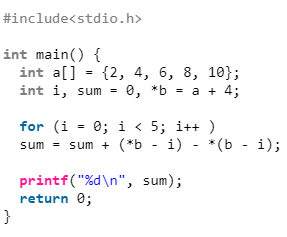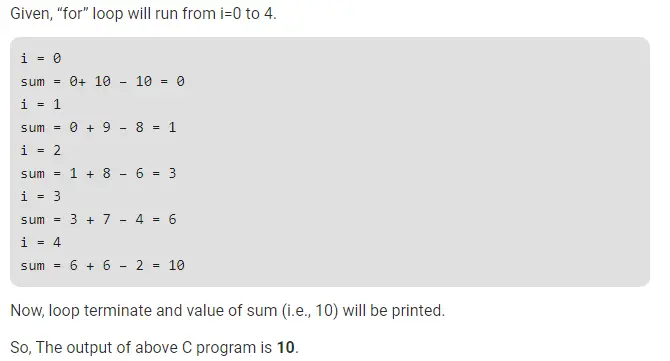# Gate CS-2019 Question Paper With Solutions

Q. 64 Consider the following C program:

``#include<stdio.h>  ` ` ` `int` `main() {  ` `  ``int` `a[] = {2, 4, 6, 8, 10};  ` `  ``int` `i, sum = 0, *b = a + 4;  ` ` ` `  ``for` `(i = 0; i < 5; i++ )  ` `  ``sum = sum + (*b - i) - *(b - i);  ` ` ` `  ``printf``(``"%d\n"``, sum);  ` `  ``return` `0;  ` `}  ``

The output of above C program is __________ .

Note: This was Numerical Type question.

(A) 10

(B) 8

(C) 6

(D) 3